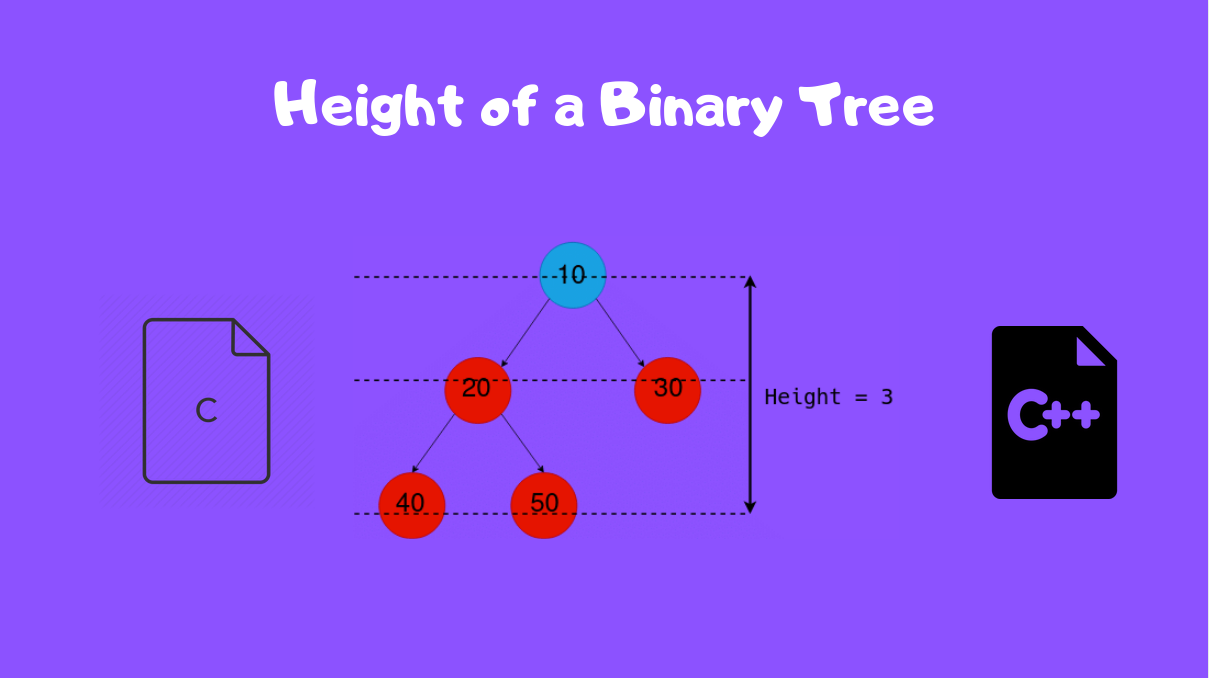# Height of Binary Tree in C/C++

Published on August 3, 2022By Vijaykrishna RamWhile we believe that this content benefits our community, we have not yet thoroughly reviewed it. If you have any suggestions for improvements, please let us know by clicking the “report an issue“ button at the bottom of the tutorial.

The height of a Binary Tree is defined as the maximum depth of any leaf node from the root node. That is, it is the length of the longest path from the root node to any leaf node.

Let us consider the below Binary Tree.

Since the leaf nodes corresponding to the maximum depth are 40 and 50, to find the height, we simply find the number of edges from the root node to either one of these two nodes, which is 3.

Now that we know what the height of a Binary tree signifies, we shall now construct an algorithm to find the height of any Binary Tree.

## Logic for finding the Height of Binary Tree in C++

Let us now decide the logic behind finding the height and write our pseudo code first.

We shall use recursion on the tree, to find the height. (Refer to the Wikipedia article for the concepts)

Since the height of the tree is defined as the largest path from the root to a leaf, we can recursively compute the height of the left and right sub-trees.

We can apply the definition of the height on the sub-trees now.

We observe that it is the maximum between the left and the right sub-trees and then add one.

Since the height of the tree is the maximum height of the sub-tree + 1, we keep doing this, until the sub-tree becomes `NULL`, and it’s height is 0.

At this point, our function will finally terminate, and

Let’s write the function `tree_height()` that computes the height.

``````// Find height of a tree, defined by the root node
int tree_height(Node* root) {
if (root == NULL)
return 0;
else {
// Find the height of left, right subtrees
left_height = tree_height(root->left);
right_height = tree_height(root->right);

// Find max(subtree_height) + 1 to get the height of the tree
return max(left_height, right_height) + 1;
}
``````

Line #3 evaluates the terminating condition, when the sub-tree size is 0, or when the root node is `NULL`.

Lines 7 and 8 recursively find the height of the left and right sub-trees.

And finally, Line 11 returns the maximum among the two, returning the height of the tree.

## Implementation in C/C++

The below is a complete program showing how the Binary Tree is constructed, and then shows the logic for finding the height in `tree_height()`.

``````#include <stdio.h>
#include <stdlib.h>

typedef struct Node Node;

// Define the Tree Node here
struct Node {
int value;
// Pointers to the left and right children
Node* left, *right;
};

Node* init_tree(int data) {
// Creates the tree and returns the
// root node
Node* root = (Node*) malloc (sizeof(Node));
root->left = root->right = NULL;
root->value = data;
return root;
}

Node* create_node(int data) {
// Creates a new node
Node* node = (Node*) malloc (sizeof(Node));
node->value = data;
node->left = node->right = NULL;
return node;
}

void free_tree(Node* root) {
// Deallocates memory corresponding
// to every node in the tree.
Node* temp = root;
if (!temp)
return;
free_tree(temp->left);
free_tree(temp->right);
if (!temp->left && !temp->right) {
free(temp);
return;
}
}

int tree_height(Node* root) {
// Get the height of the tree
if (!root)
return 0;
else {
// Find the height of both subtrees
// and use the larger one
int left_height = tree_height(root->left);
int right_height = tree_height(root->right);
if (left_height >= right_height)
return left_height + 1;
else
return right_height + 1;
}
}

int main() {
// Program to demonstrate finding the height of a Binary Tree

// Create the root node having a value of 10
Node* root = init_tree(10);

// Insert nodes onto the tree
root->left = create_node(20);
root->right = create_node(30);
root->left->left = create_node(40);
root->left->right = create_node(50);

// Find the height of the tree
int height = tree_height(root);
printf("Height of the Binary Tree: %d\n", height);

// Free the tree!
free_tree(root);
return 0;
}
``````

Thanks for learning with the DigitalOcean Community. Check out our offerings for compute, storage, networking, and managed databases.Vijaykrishna Ram

author

#### Still looking for an answer?

Ask a questionSearch for more help

JournalDev
DigitalOcean EmployeeJuly 11, 2020

in your example based on what you said “Since the leaf nodes corresponding to the maximum depth are 40 and 50, to find the height, we simply find the number of edges from the root node to either one of these two nodes, which is 3.” height of the tree is 2, not 3, right? because there are two edges from the root to 40 or 50. Can you please confirm?

- Mohsen Vafa

Click below to sign up and get \$200 of credit to try our products over 60 days!

### Popular Topics

##### Join the Tech Talk##### Hollie's Hub for Good

Working on improving health and education, reducing inequality, and spurring economic growth? We’d like to help.